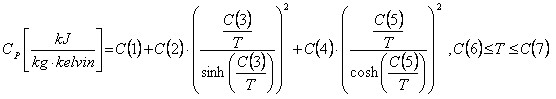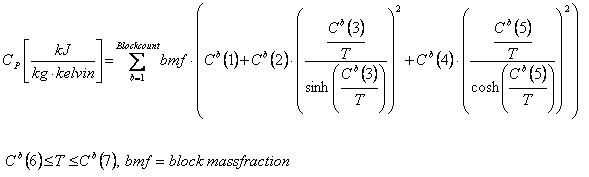﻿ Ideal gas heat capacity

# Ideal gas heat capacity

Top  Previous  Next

A number of physical properties can be calculated from an equation of state combined with a model for an ideal gas heat capacity.

VLXE output the most common:

Enthalpy

Entropy

The two most common expressions for the ideal gas heat capacity in VLXE are:

Polynomial

DIPPR

The user sets the model independent for solvents and polymers.

Reference temperature and pressure

When calculating e.g. enthalpy and entropy a reference temperature and pressure is needed in the integration of the ideal gas expression,

The values used in VLXE are:

Reference temperature: 298.15 Kelvin

Reference pressure: 1.0 bar

Polynomial

The polynomial is given as:Note the units of Cp.

C(7) and C(8) defines the temperature range where the expression is valid

For co-polymer the formula used is:DIPPR

The DIPPR expression is given by:Note the units of Cp.

C(7) and C(8) defines the temperature range where the expression is valid

For co-polymers the formula used is:Often the parameters used are reported as dimensionless values,however it is converted into units as:Note that in the DIPPR expression only C1, C2 and C4 has to be converted.

Poly disperse polymers

Polymers that are poly disperse poses a special problem since the parameters for the ideal heat capacity expression cannot be given for each pseudo component and often is not known.

The method that is used in VLXE is based on the fact that for heavy alkane's the value for Cp on a mass basis becomes independent on the alkane as the molar mass becomes big.

This information is used, so only one set of parameters has to be given for a polymer. The parameters are then scaled using the molar mass of each pseudo component.

Parameters for a given polymer mono-/poly-disperse

If no values are available for a given polymer it is recommended to use the values for a C20H42: Eicosane.

The values are:

 C(1) C(2) C(3) C(4) C(5) C(6) C(7) C(8) Polynomial Cp(kJ/kg K) 8.1694E-1 -3.0569E-4 1.5706E-5 -2.1058E-8 8.5058E-12 0 200 1000 DIPPR    Cp  (kJ/(kg K) 1.1496 3.9249 1636.0 2.6367 -726.27 200 1500 -

Not much research has been done in this field. As more research is done VLXE will become updated.